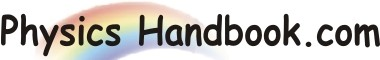HOME TOPICS DEFINITIONS TABLES LAWS INVENTIONS EXPERIMENTS QUIZ VIDEOS
 Wien's Displacement Law The Wien's Displacement Law state that the wavelength carrying the maximum energy is inversely proportional to the absolute temperature of a black body. i.e λmax x T = b Where, λmax  =  Wavelength of maximum intensity ( meters ) T  =  Temperature of the blackbody ( kelvins ) b  =  Wien's displacement constant  =  2.8977685 ± 51 × 10-3 meters·kelvins For optical wavelengths, it is often more convenient to use the nanometer in place of the meter as the unit of measure. In this case, b  =  Wien's displacement constant  =  2.8977685 ± 51 × 106 nm·K The law is named after German physicist Wilhelm Wien.